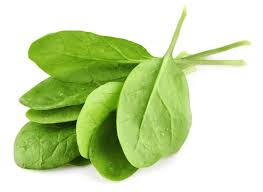# I am spinach $__$

Probability Level 3There are $\color{limegreen}{\textbf{17 raw spinach leaves}}$ in a $\color{#3D99F6}{\text{Blue bag}}$ and $\color{#20A900}{\textbf{19 ripe spinach leaves}}$ in a $\color{#D61F06}{\text{Red bag}}$.

I want to put the $\color{#3D99F6}{\text{Blue bag}}$ and the $\color{#D61F06}{\text{Red bag}}$ in a $\color{#69047E}{\text{Purple bag}}$ after removing at least one (and maximum all) leaves from each of the 2 bags.

Then how many different possibilities are there for the contents of the $\color{#69047E}{\text{Purple bag}}$ ?

Details and assumptions:-

$\bullet$ The contents of the $\color{#69047E}{\text{Purple bag}}$ are counted in terms of $\color{#D61F06}{(Red,}\color{#3D99F6}{Blue)}$.

$\bullet$ If i remove 3 leaves from $\color{#3D99F6}{\text{Blue bag}}$ and 4 leaves from the $\color{#D61F06}{\text{Red bag}}$, then the $\color{#69047E}{\text{Purple bag}}$ will have the contents $\color{#D61F06}{(Red,}\color{#3D99F6}{Blue)} = (15,14)$.

($\color{limegreen}{3}$ out of $\color{limegreen}{\text{17 raw leaves}}$ and $\color{#20A900}{4}$ out of $\color{#20A900}{\text{19 ripe leaves}}$ were removed)

This problem is part of the set Vegetable Combinatorics

×

Problem Loading...

Note Loading...

Set Loading...